# Inviscid Flow

Inviscid flow is a schematic representation of the motion of mobile media (gaseous or liquid, and also solids under the rapid action of high pressures). It is the main theoretical model for many fields of modern technology. Calculation results obtained within the framework of this model are widely used in designing flying vehicles, rockets and their engines, turbines and compressors; in analyzing the motion of shells in the bore of a gun and their trajectories in the atmosphere; in designing the combustion and detonation of fuels and explosives; in determining the action of explosive waves on barriers; in describing high-speed collisions of solids; and in many other cases.
Studies of inviscid flows are carried out in gas dynamics, acoustics, electro- and magneto-gas dynamics, the dynamics of rarefied gases, plasma dynamics, etc. In the theory of rarefied gases and plasma a statistical description of the behavior of fluid particles comprising the medium is used. In other cases, the flow may be considered to be within the scope of the continuous medium model with the use of small, volume-averaged values of mass, momentum and energy.
If the viscosity and thermal conductivity of a fluid are ignored, its velocity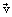, pressure P and density ρ (at points in space where they are continuous) must be related by the following equations: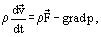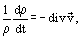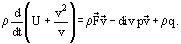The first equation, Eider's equation, relates the acceleration of a fluid particle (i.e., an elemental volume of fluid) to an external body force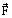and the pressure force applied on the side of the neighboring fluid particles. This equation is the generalization of Newton's second law (the law of conservation of momentum) as applied to the motion of fluid particles.
The second equation expresses the law of mass conservation: the rate of change of density of a fluid particle is equal, with the sign reversed, to the rate of change of volume. The third equation is the law of energy conservation: a change in the internal and kinetic energy of a fluid particle is due to an action of impressed mass forcesand surface forces (pressure p), and to an inflow of heat with intensity q from an external source.
A strong heat release inside a moving gas or an inflow of heat from the outside can cause considerable changes in density. The compressibility of a medium is the principal feature of many problems which can be solved with the help of the inviscid flow model. This, in turn, calls for a wider application of thermodynamics (see Thermodynamics, Irreversible Thermodynamics, Multicomponent Systems, Thermodynamics of) and the inclusion of the equation of medium state into the system of defining equations (see Equations of State, PVT Relationships).
In a number of problems, the equations for an inviscid flow must be supplemented with kinetic equations to derive the rates of internal physicochemical transformations, chemical reactions between the components of a mixture, dissociation or ionization, and also for the excitation of internal degrees of freedom, etc.
The parameters of gas can undergo sudden changes on some surfaces inside the flow area. There are two types of discontinuity surfaces. If no flow passes through a discontinuity surface, then this is the tangential discontinuity surface (for instance, in parallel flow of two gases which differ in density, temperature and in velocity). A typical example of a discontinuity surface of the second type is a shock wave (see Waves in Fluids). In this case the flow of fluid particles intersects the discontinuity surface, and the equations for finite volume of gas are written in integral form. By geometrical criteria, problems solved with the help of an inviscid flow model are subdivided into internal and external problems. Internal flows refer to flows in nozzles, in blades of gas turbines, in aerodynamic wind tunnels, etc. (see Nozzles, Turbines, Wind Tunnels). If a streamlined body is immersed in a liquid whose velocity at infinity is described by a uniform profile, then such a problem refers to a class of external inviscid flows (see External Flows, Overview).
The most important fluids, air and water, have significant (though rather low) coefficients of viscosity. But even for them, the inviscid flow model gives a good description of real processes at some distance from bounding solid surface. A more rigorous statement of the problem necessitates the use of the Navier-Stokes equations for fluid motion which, unlike Euler's equations, allow for internal friction or tangential stresses between neighboring layers and also for the molar thermal conductivity of the medium. Although, with the Reynolds number Re tending to infinity, the Navier-Stokes equations become at the limit the Euler ones, a drop in the order of the differential equations (from the fourth to the second order) occurs. Therefore, the solution of simplified equations will not satisfy the boundary conditions of a complete system of equations. It can also be supposed that in the limiting case of Re → ∞, the solutions for the Navier-Stokes equations contain the assumption that the entire field of flow can be divided into two areas: a thin layer area (see Boundary Layers) which surrounds the body and propagates behind it in the form of a narrow wake; and an external flow area separated from the body by the said layer. It is a known fact that in a number of cases such a division is wrong; for instance, in the case of separation of the boundary layer.
The purpose of solving all the above-mentioned problems with an inviscid flow model is to determine the impact of the moving fluid on a streamlined body. Sometimes, the total field of gas-dynamic parameters (velocity, density, temperature) must be known in the entire flow or in some specified sections (at the nozzle cut, at the outer edge of the boundary layer, etc.).
Solutions to a system of equations of an inviscid flow are usually obtained with some simplification. The first simplification method reduces the number of independent variables. Cases of similitude and self-similarity of flows are of some interest. For instance, in describing strong explosions in half-space and also in the propagation of flame fronts or detonation caused by cylindrical (plane) sources, the dimensionless values of the gas parameters behind the shock wave can be described by a system of ordinary differential equations in one self-similar variable: ξ = E0t2/(ρ1r2+v) (where E0 is the energy of explosion released immediately at the moment t = 0; ρ1 is the initial density of the gas medium; r is the distance from the explosition site; v = 3 for spherical, v = 2 for cylindrical and v = 1 for plane shock waves).
Among stationary inviscid flows, the self-similarity model is typified by a homogeneous supersonic flow moving around an infinite thin cone (Figure 1). It is impossible to distinguish a typical linear dimension in this problem. Extending or compressing the flow field around the cone vertex an arbitrary number of times does not change the picture—i.e., it remains similar to itself. In an axially-symmetric flow around the cone, fluid flow is retarded first in a conical shock wave (OS) trailing at the cone vertex, and then in a compressed layer of gas present between a shock wave and the body; the flow is turned to a direction corresponding to the cone surface (the streamline "a").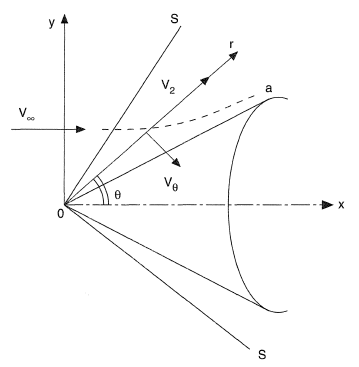Figure 1. Homogeneous supersonic flow around an infinite thin cone.
If a shift is made from the Cartesian system x, y to a polar coordinate system r, θ and if we denote by vr and vθ the components of the velocity vector, then the only self-similar coordinate will be the polar angle θ. The equations of an inviscid flow are reduced in this case to a system of two ordinary differential equations: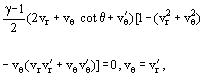where γ = cp/cv is the ratio of specific heat capacities of the gas, and the prime means differentiation with respect to θ.
Self-similarity, in the broad sense, sometimes means the independence of dimensionless parameters characterizing the flow from similarity criteria (see External Flows, Overview and Drag Coefficient).
The most important results of theoretical analysis of inviscid flows have been obtained for stationary and irrotational flows. The acceleration of a fluid particle, entered into the Euler equation, can be written as follows: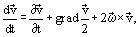where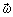= 1/2 curlis the velocity vector curl. For a steady-state flow (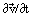= 0), when= 0 or||, the Euler equation allows a general integral. Since the mass forces have a potential in a majority of cases—i.e., can be written as= −grad Φ, where Φ is the mass force potential or the force function—the general integral of Euler's equation takes the form known as Bernoulli's integral: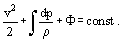For a heavy incompressible fluid Φ = gZ, and ρ = const,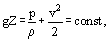where Z is the vertical coordinate.
For weightless, incompressible fluid,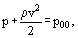where p00 is the pressure of the stagnated flow (or impact pressure). The second term on the left-hand part of the equation is called velocity or dynamic head.
For the isothermal motion of compressible gas p/ρ = const, Bernoulli's integral is written as follows: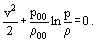Finally, the equation of state of the adiabatic process p/ργ = const allows Bernoulli's integral to be written as: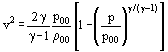(notation of this kind was suggested by De Saint Venant et Wantzel in 1839).
The idea of irrotational motion seems quite fruitful not only because Bernoulli's integral exists in this case but it also makes it possible to obtain a simple relation between the modulus of the fluid flow velocity vector and the pressure. Also, an important point here is that three unknown quantities—the projections of speed onto the coordinate axes, Vx, Vy and Vz—can be expressed in terms of one unknown function, the velocity potential φ(x, y, z).
The vector equation= 1/2 curl= 0 in projections onto the coordinate axes is equivalent to the fact that there exists a certain function φ(x, y, z) whose partial derivatives with respect to x, y, and z are equivalent to the appropriate velocity components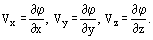If the mass force≡ 0 is neglected, then a single scalar equation for velocity potential, instead of the Euler vector equation, can be written: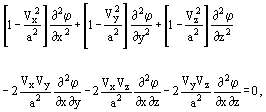where a2 = (dp/dρ) is the sound velocity in gas.
Depending on whether the motion is subsonic or supersonic, the differential equation is an elliptic or hyperbolic one. Inviscid flows of an incompressible fluid form a large and important class because with the velocity of flow much lesser than the velocity of sound, the velocity potential equation takes the form of the Laplace linear equation, which is well-studied in mathematics: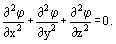The set of its solutions forms the class of Harmonic Functions.
The solution of two-dimensional (plane) problems has been completed with the use of the theory of functions of complex variables. Any potential plane-parallel flow of inviscid gas can be described by a complex potential W = φ − iψ, where φ(x, y) and ψ(x, y) are two harmonic, mutually conjugate functions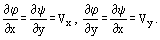The function ψ(x, y) has a simple hydrodynamic meaning: substituting into the streamline equation (see Streamline)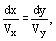the value of the velocity projections expressed in terms of the function ψ yields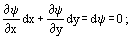hence, it follows that the function ψ(x, y) preserves a constant value along the streamlines ψ = const (see Stream Function).
It is easy to verify that a family of isopotential lines φ(x, y) = const and a family of streamlines ψ(x, y) = const are mutually orthogonal, i.e., they intersect at a right angle (Figure 2).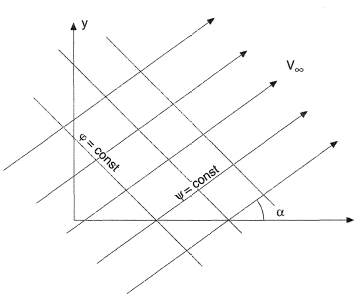Figure 2. Orthoganality of isopotential lines (φ(x, y) = constant) and streamlines (ψ(x, y) = constant).
Each of the two conjugate functions φ and ψ can be interpreted as the velocity potential or the current function. This procedure is usually used in designing the flows.
Introducing a complex potential W allows a decrease in the order of the equation: instead of an unknown function of two variables ψ(x, y) the function of one complex variable W(Z) must be found, where z = x + iy is in Cartesian coordinates and Z = re is in polar coordinates.
By way of conclusion, some simple functions of a complex variable Z are considered below and a geometric interpretation of flows they describe is provided. Figure 2 shows a homogeneous flow with velocity V and inclined to the real axis of a physical plane at an angle α described by a linear function W = VZ. Source and run-off flows, described by logarithmic functions (A > 0 for the source and A < 0 for the run-off), are depicted in Figure 3. Finally, Figure 4 shows a homogeneous flow with velocity V past a cylinder with radius a, obtained as the sum of a linear and a reverse function W = |V|(Z + a2/Z).
As the last example shows, the superposition principle is of prime importance for potential flows when the flow with the potential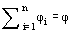is obtained out of several simple flows with potentials φi. The velocity vectors of integrable flows at each point are added vectorially.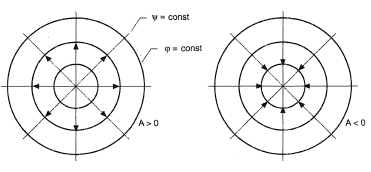Figure 3. Source and run-off flows.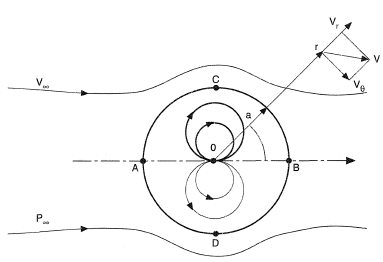Figure 4. Flow around an infinite cylinder.
This procedure manifests itself most eloquently for a plane potential flow. If the streamline configurations of two integrable plane flows are known then their superposition on one drawing forms a grid which makes it possible to draw the streamlines of the resultant flow. The flow around an infinite cylinder of radius a with velocity V, (Figure 4) is the sum of the plane homogeneous flow parallel to the OX axis with complex potential W1 = |V|Z, and of the velocity field dipole with a complex potential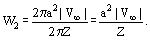The dipole is the limiting set of the source and the run-off when the distance r between them tends to zero, and the power Q increases up to infinity so that the product m = Q · r = const.
The flow picture in Figure 4 consists of two areas: outside and inside the circle of radius a. The first area |Z| ≥ a, is the external flow around the cylinder. The second area, |Z| ≤ a, is the flow formed by the dipole inside the circle of radius a. The velocity modulus on the circle contour varies according to the sine law |V| = 2|V| sin θ. The points A (θ = π) and B (θ = 0) are called the front and rear critical points, respectively, or the branching points of the flow.
The velocity on the cylinder surface assumes its maximum value at points C and D, which correspond to the mid-section θ = ± π/2. It is interesting to note that the maximum velocity on the cylinder contour exceeds the velocity of the free-stream flow by a factor of 2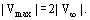The distribution of static pressure on the cylinder surface can be easily obtained from the known distribution of velocity. For this purpose, Bernoulli's integral can be used and the result can be represented in dimensional form with the help of a pressure coefficient Cp: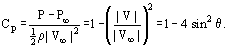Such a representation of pressure is convenient because Cp is independent either of fluid density ρ, or of pressure or velocity at an infinite distance from the cylinder, p or V. Thus, a universal form of notation for pressure distribution on a cylinder surface of any size under arbitrary conditions in a free-stream flow is obtained.
Experimentally, the measured pressure distribution starts to vary from the one calculated from inviscid flow theory even before the point of maximum velocity |Vmax| or minimum pressure p on the surface of the cylinder. The reason for this divergence is the impossibility of smooth (continuous) flow by a real fluid around a cylinder.
The cylinder and the sphere are two examples of poorly-streamlined bodies. After breaking down into two flows at the front critical point, the free-stream flow can merge again into one flow at point B only under certain conditions. Such conditions correspond to very low velocities |V| of free-stream flow and, to be more precise, to Reynolds numbers less than unity. In the general case fluid particles on both sides of the cylinder or the sphere already in the vicinity of the mid-section tend toward the trajectories parallel to the flow axis. A stagnant zone with reduced pressure relative to p is formed between them in the wake of the cylinder. The pressure difference at points A and B is the source of the resistance the bodies experience while moving in fluid or gaseous media (see Drag Coefficient). The discrepancy between potential flow around the cylinder and the real process provides an explanation of D'Alembert's paradox. For well-streamlined bodies the stagnant zone is either absent completely or is reduced to a minimum, and the calculations of pressure according to an inviscid (potential) flow model agree well with the measurements.
The effect of the flow's three-dimensionality can be analyzed by comparing the distribution of velocity and pressure on the surface of a plane (cylinder) or a three-dimensional, axially-symmetric body, a sphere. Using the superposition principle, the potential for inviscid flow over a sphere is obtained by superposing a homogeneous flow with velocity |V| onto the flow from a dipole: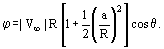Velocity distribution on the surface of a sphere is characterized by equations of the form: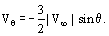The maximum value of velocity is (Vθ)max = 3/2|V|. Comparing the results for the sphere and the cylinder, note that in three-dimensional flow the deformation is much lesser and the maximum acceleration of fluid particles on the cylinder's mid-section is almost 1.35 times higher than that of the sphere.
The distribution of pressure on the surface of the sphere has the form: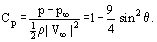Although the maximum reduction in pressure is not so great as on the cylinder, the break-off and resistance due to nonsymmetry of pressure at the critical points are also characteristics of the sphere.
Examples of flows around a sphere and a cylinder show that even at subsonic flow velocities (Mach numbers Ma < 1), the local values of velocity can be supersonic. The break-up of fluid particles that follows occurs in shock waves (see Waves in Fluids) and brings about a sharp increase in resistance, analogous to the so-called sound barrier in aviation.
Methods for solving the equations of motion and continuity equations for supersonic inviscid fluid flows have also been developed. The most widely used is the method of characteristics.
The characteristics of equations for supersonic flow, together with the gas velocity vector, form an angle at the given point equal to the angle of propagation of disturbances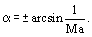At any point of space with supersonic flow, the velocity vector is directed along the bisectrix of the angle α contained by the characteristics. Projections of gas velocity from the normal to the characteristic at the given point is equal in magnitude to the local velocity of sound a.